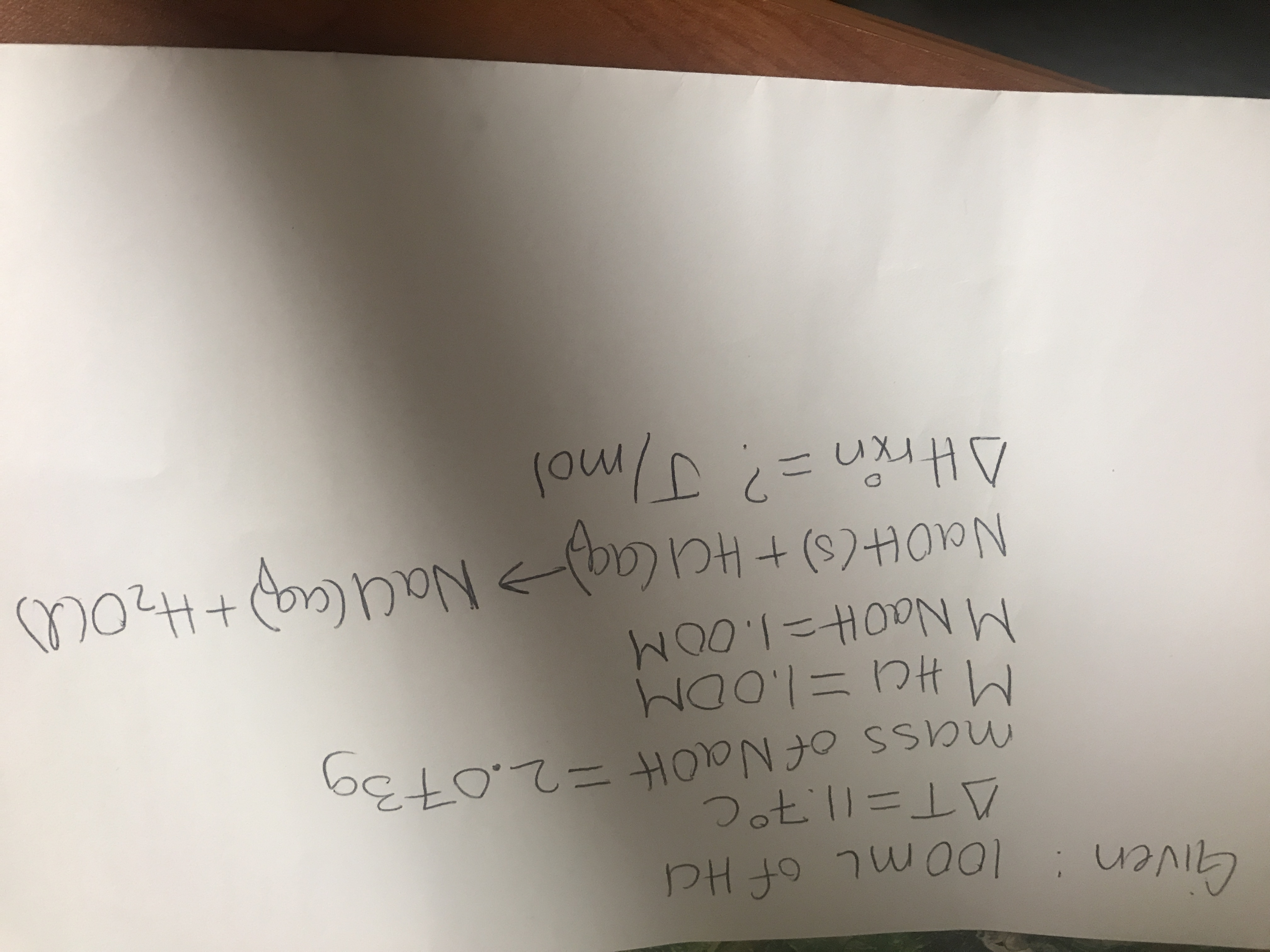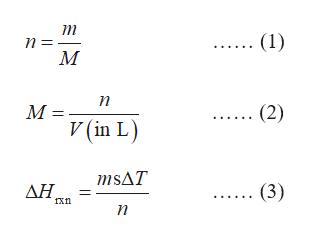# Gven: 10mL of HdDotll=mass of NaOH2.013aMHC-1.0 OMM Naot-1.0OMNaOt(s)+HCI CaqNaulaa+H2O)Atrin J/mol

Questionhelp_outlineImage TranscriptioncloseGven: 10mL of Hd Dotll= mass of NaOH2.013a MHC- 1.0 OM M Naot-1.0OM NaOt(s)+HCI CaqNaulaa+H2O) Atrin J/mol fullscreen
check_circle

Step 1

The number of moles of a species is calculated by expression (1), in which, n is the number of moles, m is the mass, and M is the molar mass of the species.

The molarity of a solution is the number of moles of solute present in one-liter solution as stated in equation (2), where, M is the molarity of solution, n is the number of moles, and V is the volume of solution in liters.

The enthalpy change of a reaction (ΔHrxn) is related to its specific heat in accordance with expression (3), in which, m is the mass of the metal, s is the specific heat, n is the number of moles of the limiting reactant, and ΔT is the change in temperature.help_outlineImage Transcriptioncloseт (1) п М п (2) М- V (in L) msAT ΔΗ, (3) n fullscreen
Step 2

The number of moles of NaOH in the given reaction is calculated using equation (1),

Step 3

The conversion factor for mL and L units is,

1 mL = 10-3 L

Hence, 100 mL is equal to 0.100 L.

The...

### Want to see the full answer?

See Solution

#### Want to see this answer and more?

Solutions are written by subject experts who are available 24/7. Questions are typically answered within 1 hour.*

See Solution
*Response times may vary by subject and question.
Tagged in

### Chemistry